# R S Aggarwal Solutions for Class 11 Maths Chapter 29 Mathematical Reasoning

R S Aggarwal Class 11 Solutions of Chapter 29 consist of various concepts on Mathematical Reasoning, explained step by step for an easy understanding. In Mathematical language, there are two kinds of reasoning, namely inductive and deductive. Mathematical induction is sort of inductive reasoning. In this chapter we will study about deductive reasoning, which contains, declarative sentences, imperative sentences, interrogative sentences and exclamatory sentences. R S Aggarwal Solutions help students to practice questions based on different such concepts. Download the detailed R S Aggarwal solutions for class 11 for Chapter 29 and score good marks in exams.

## Download PDF of R S Aggarwal Solutions for Class 11 Maths Chapter 29 Mathematical Reasoning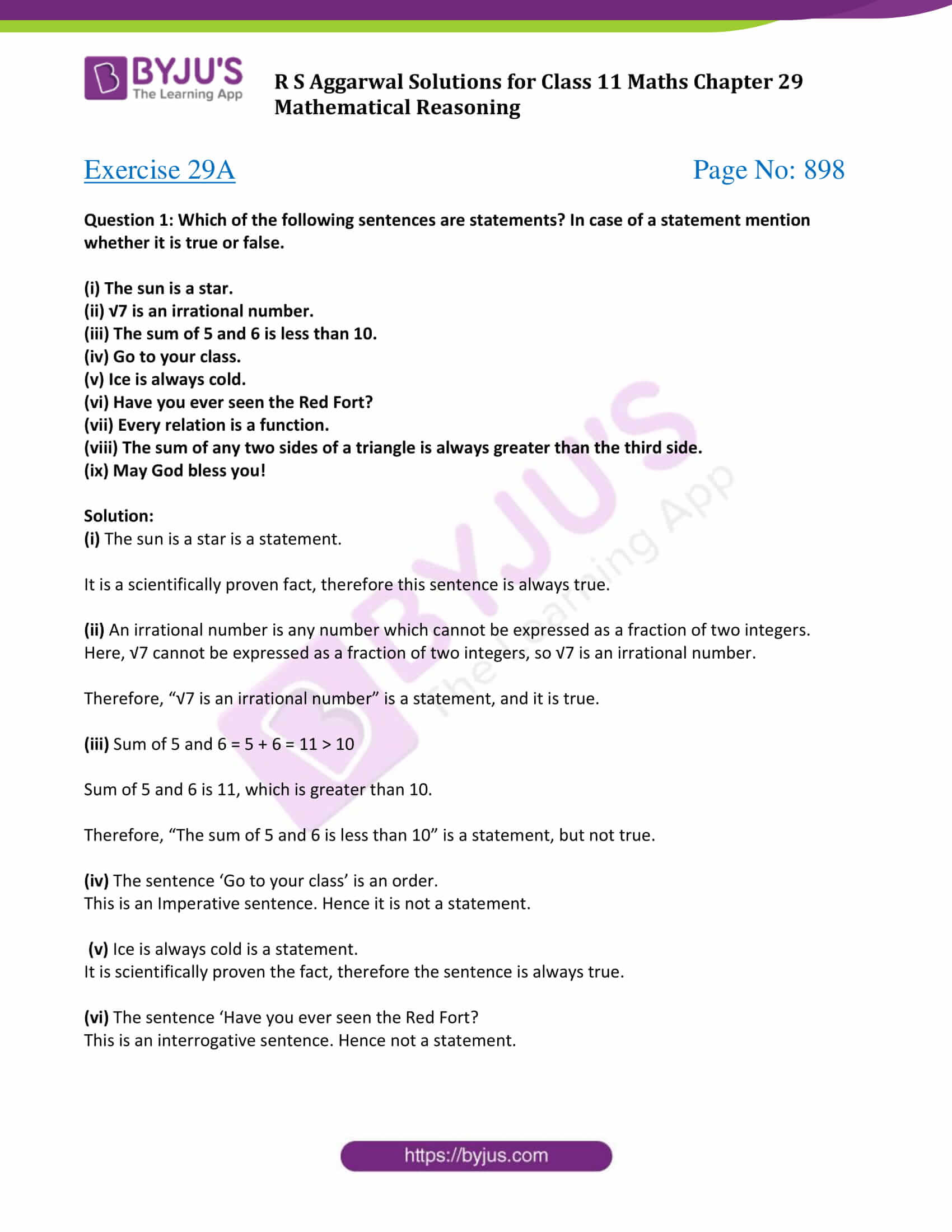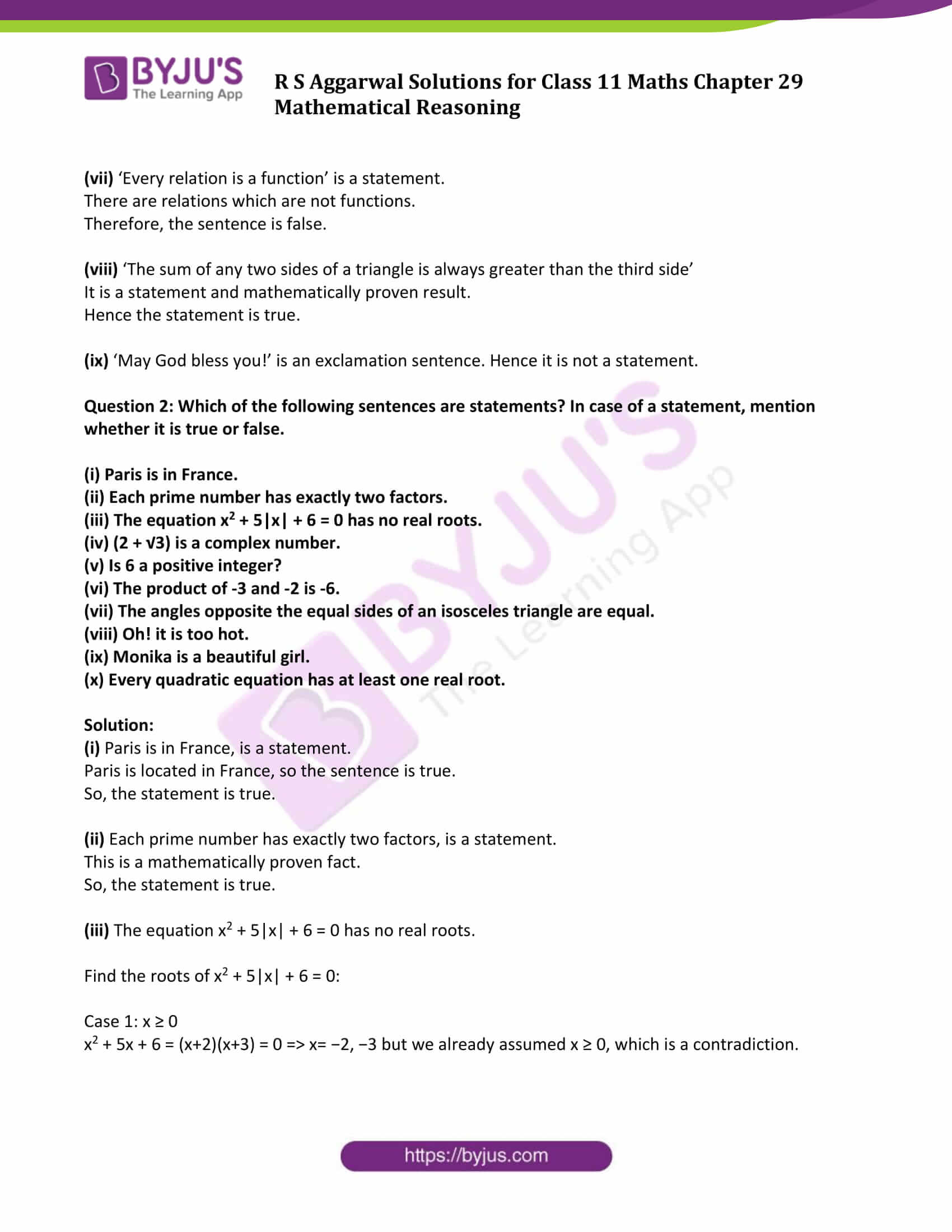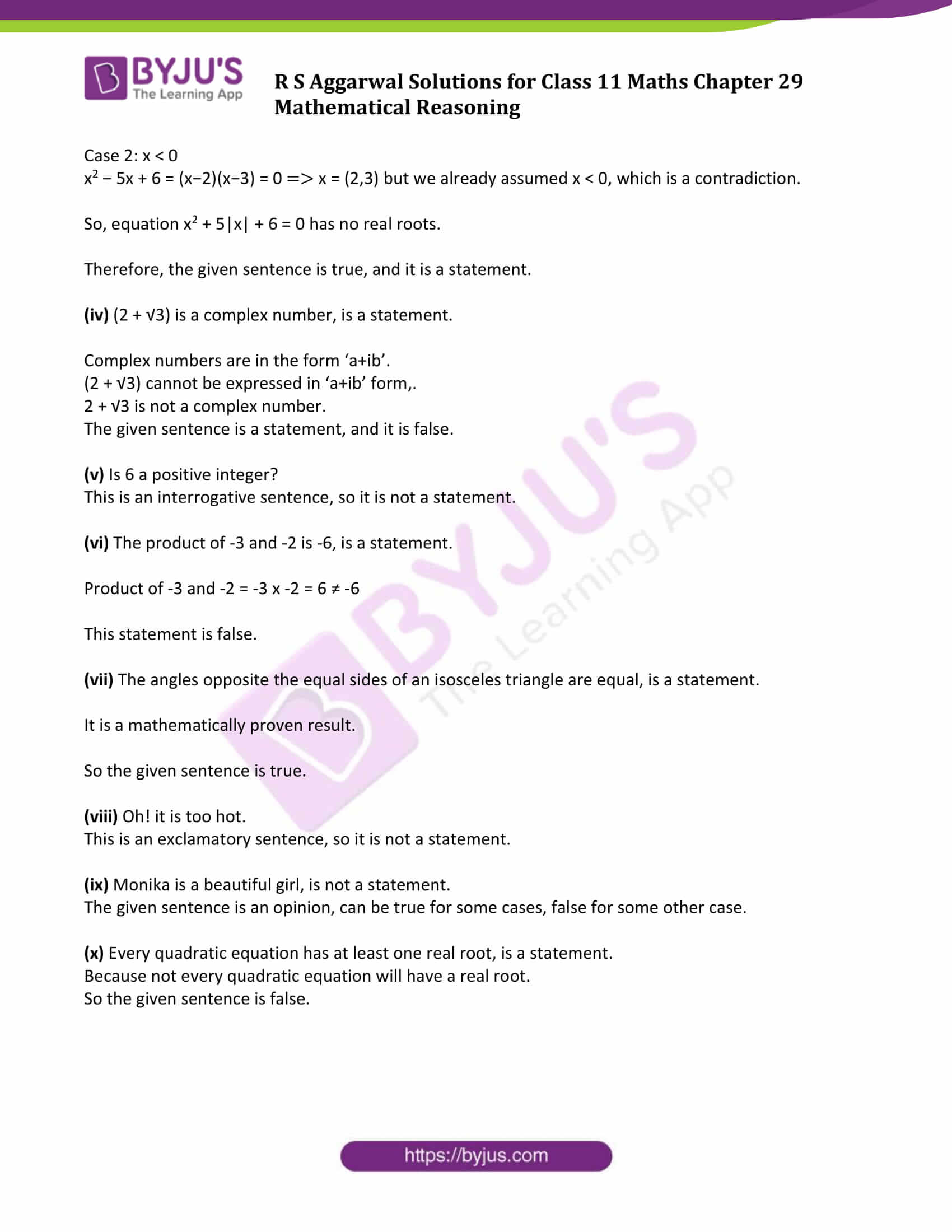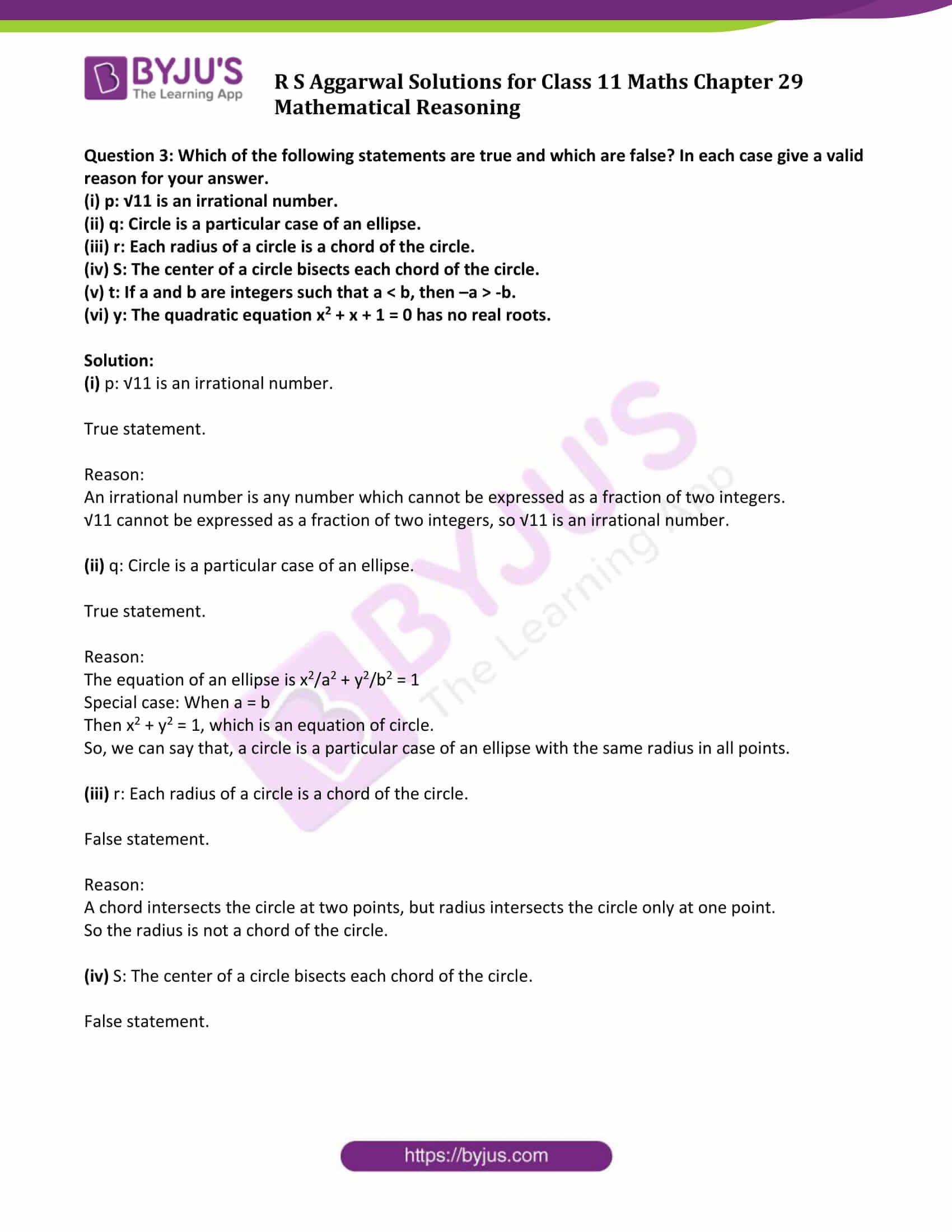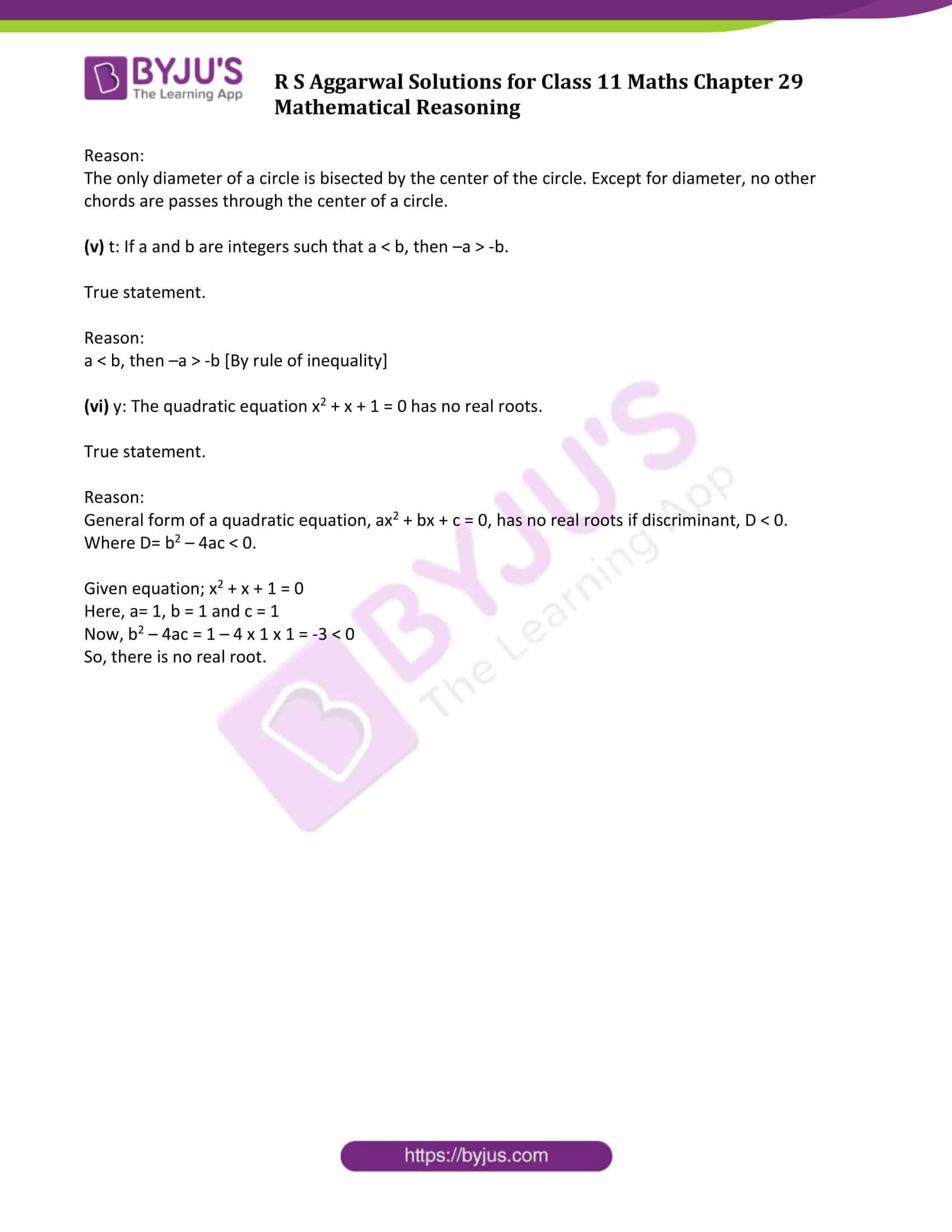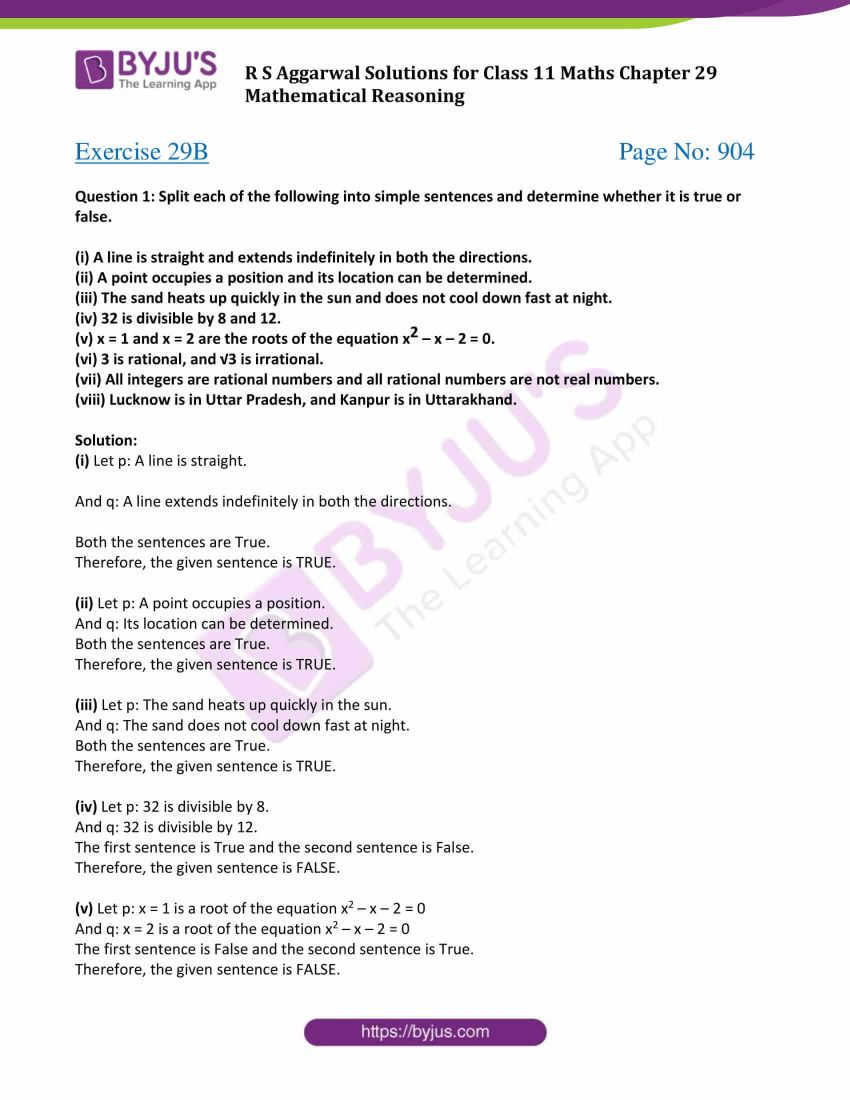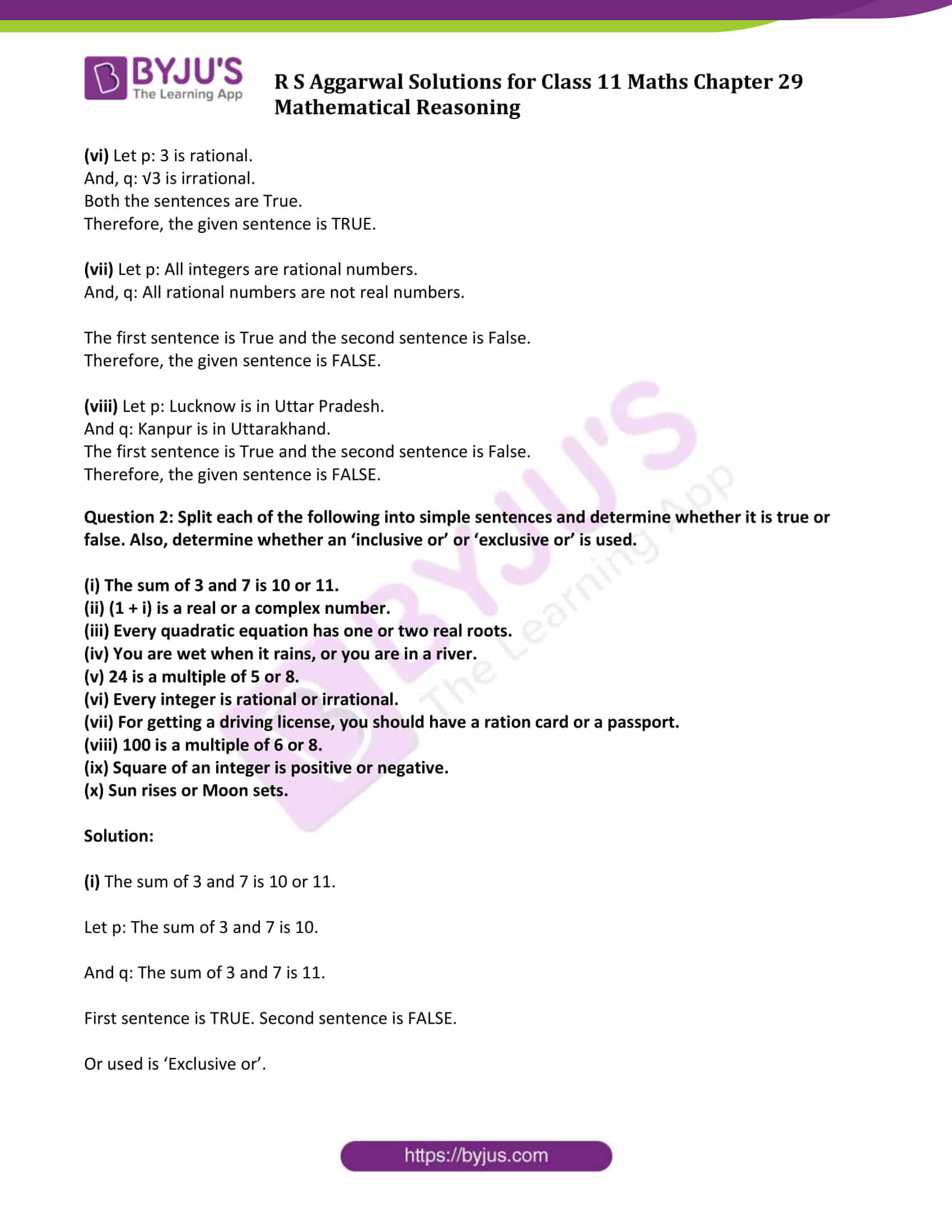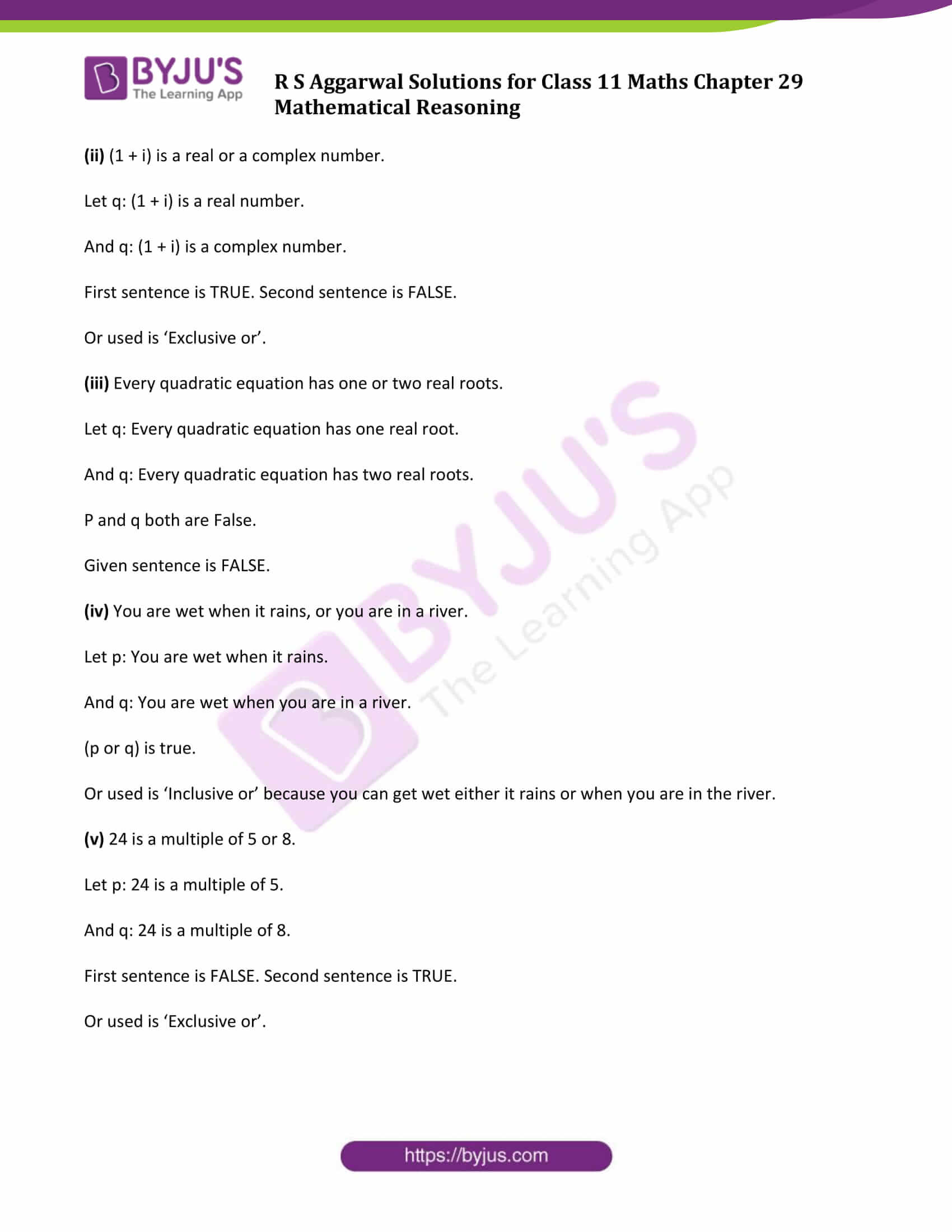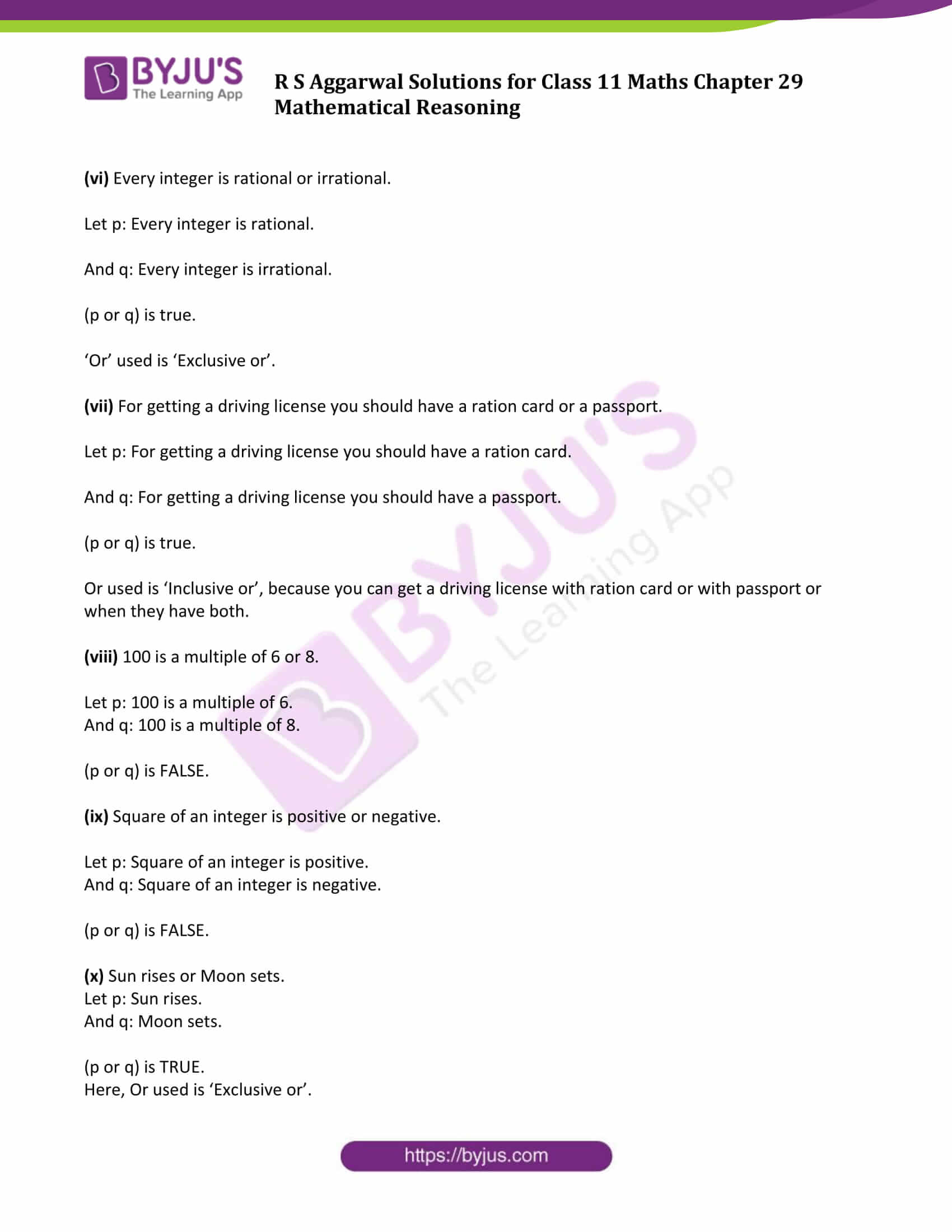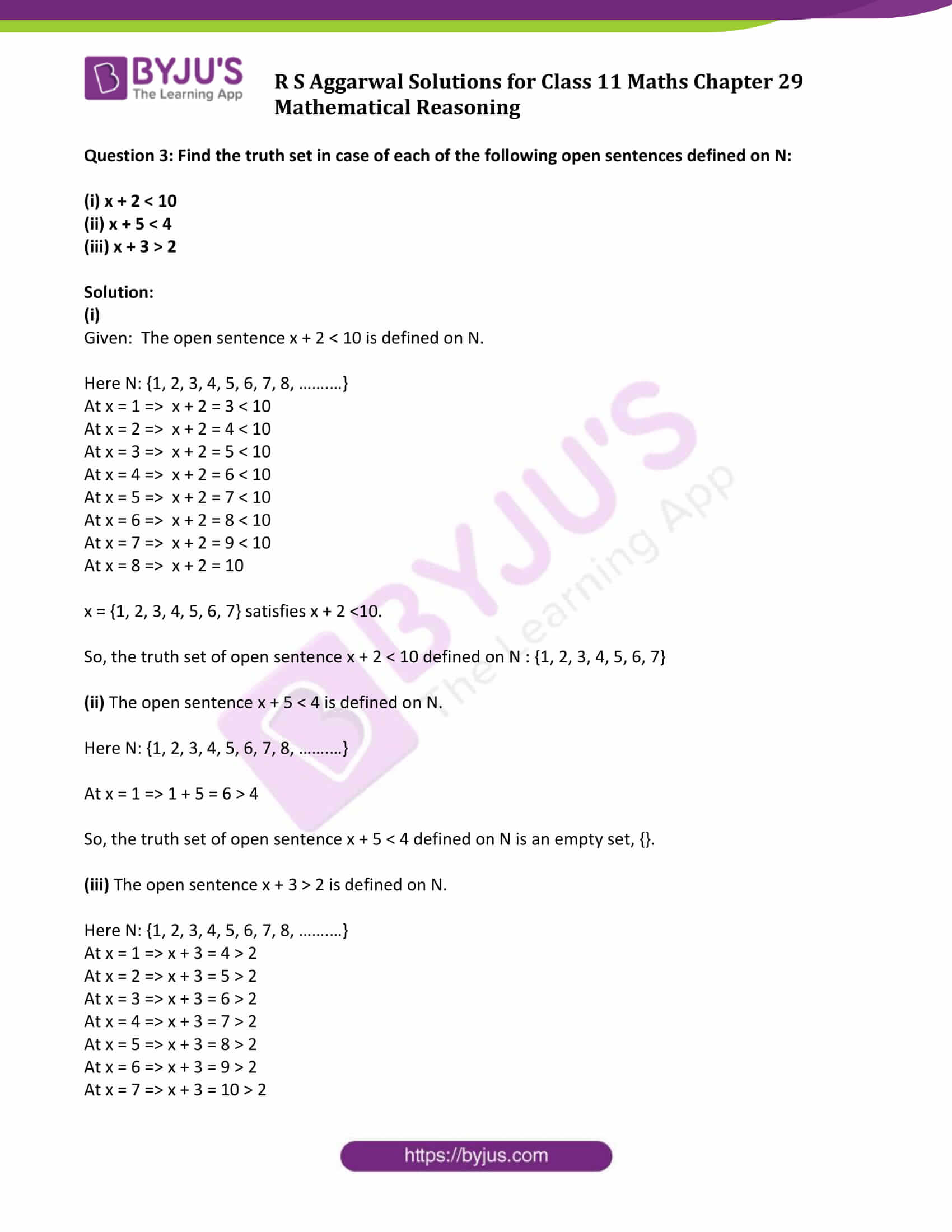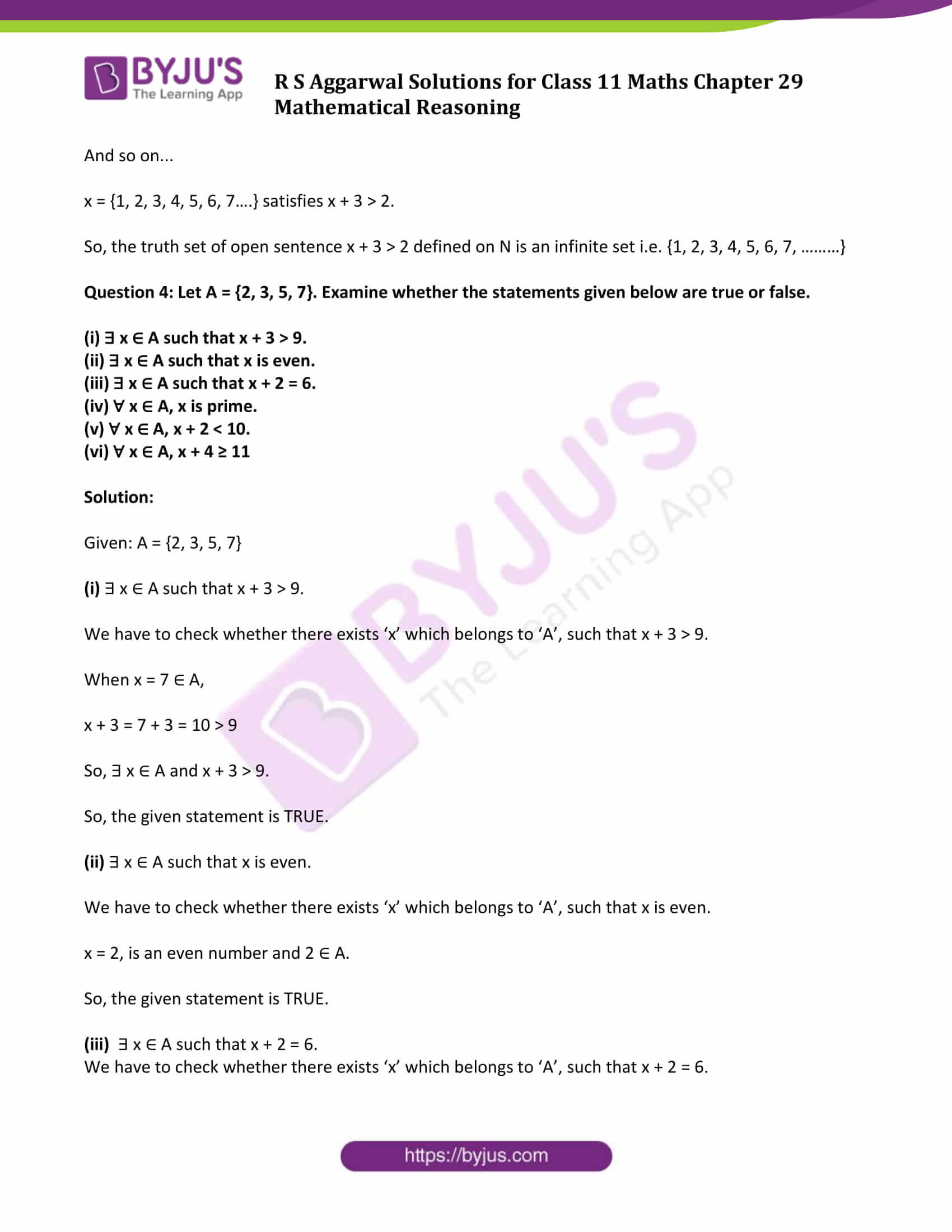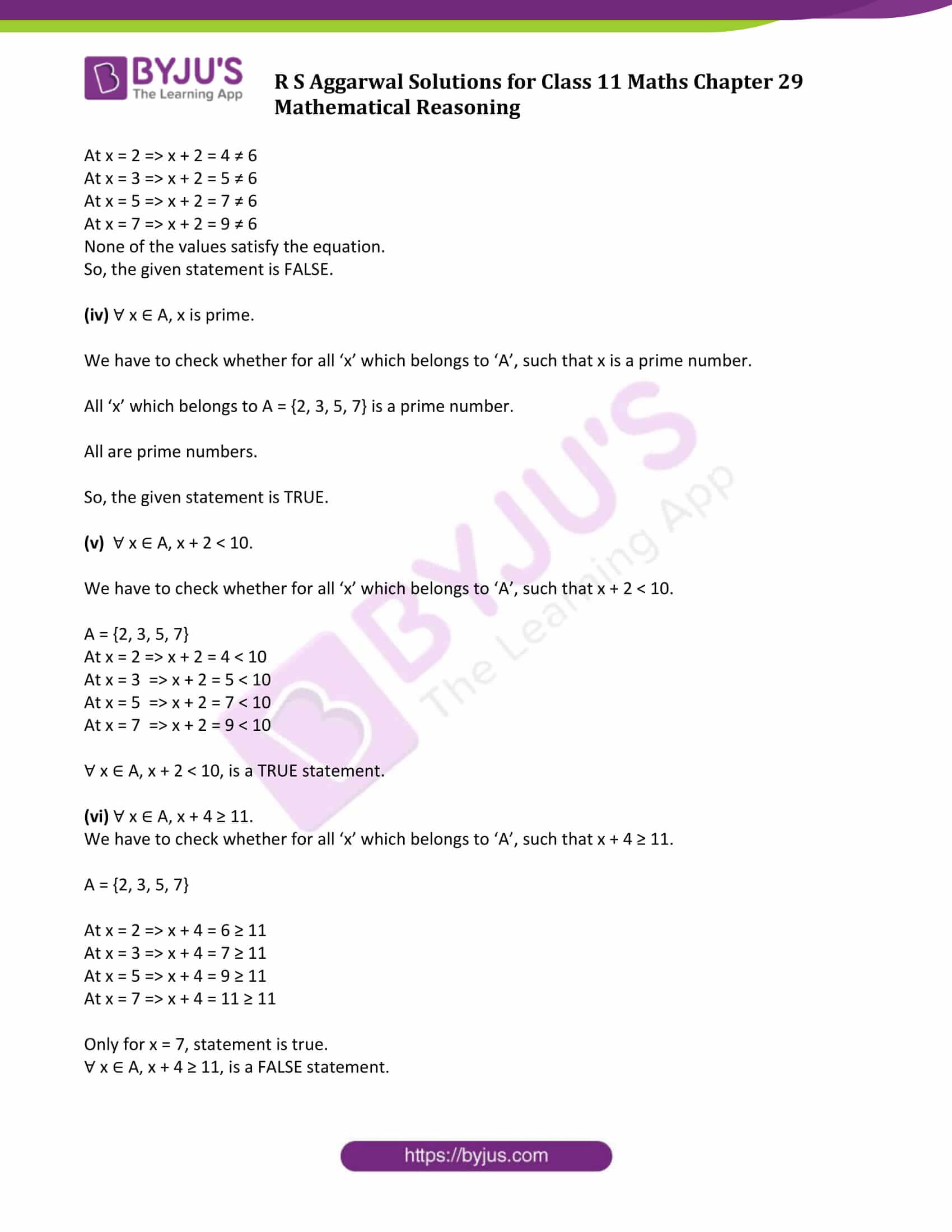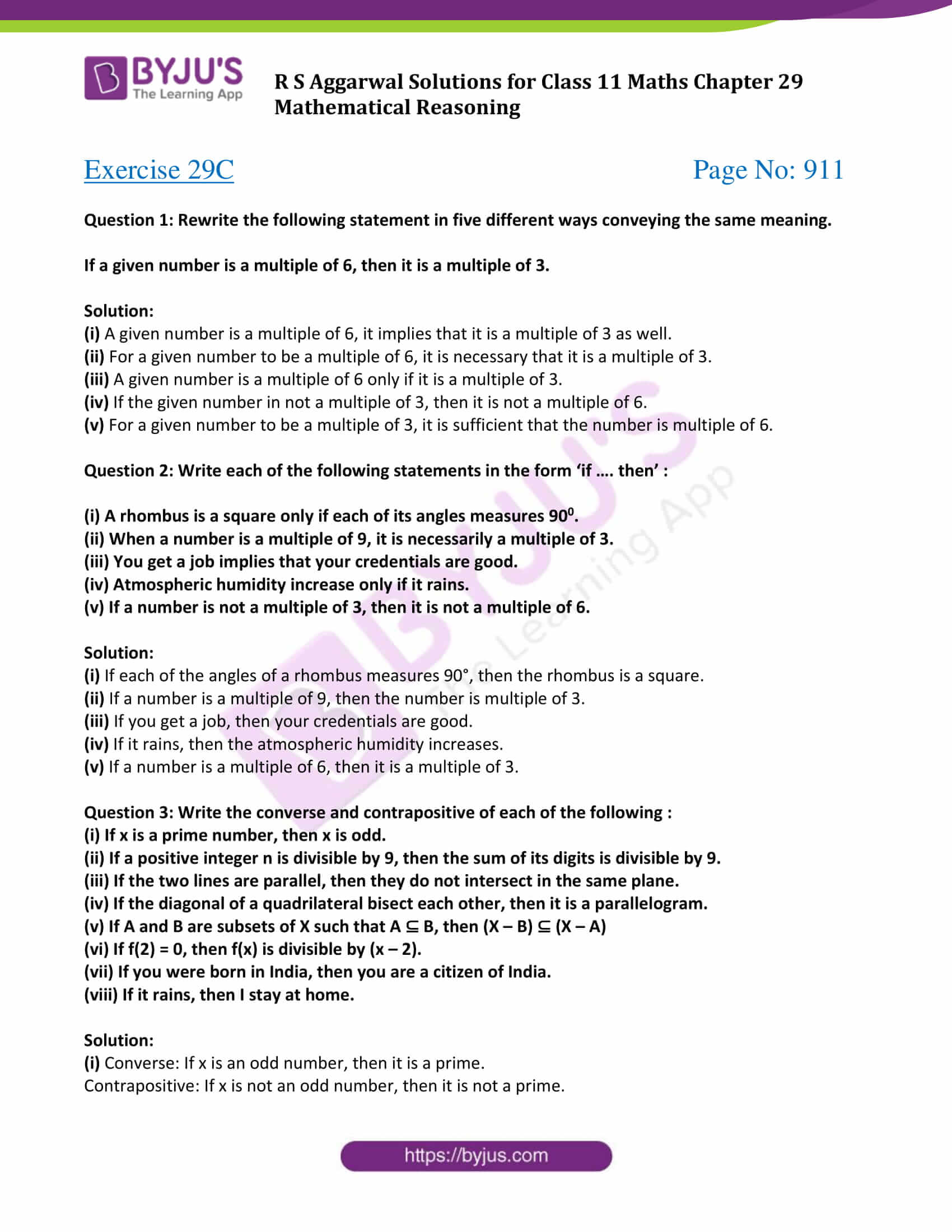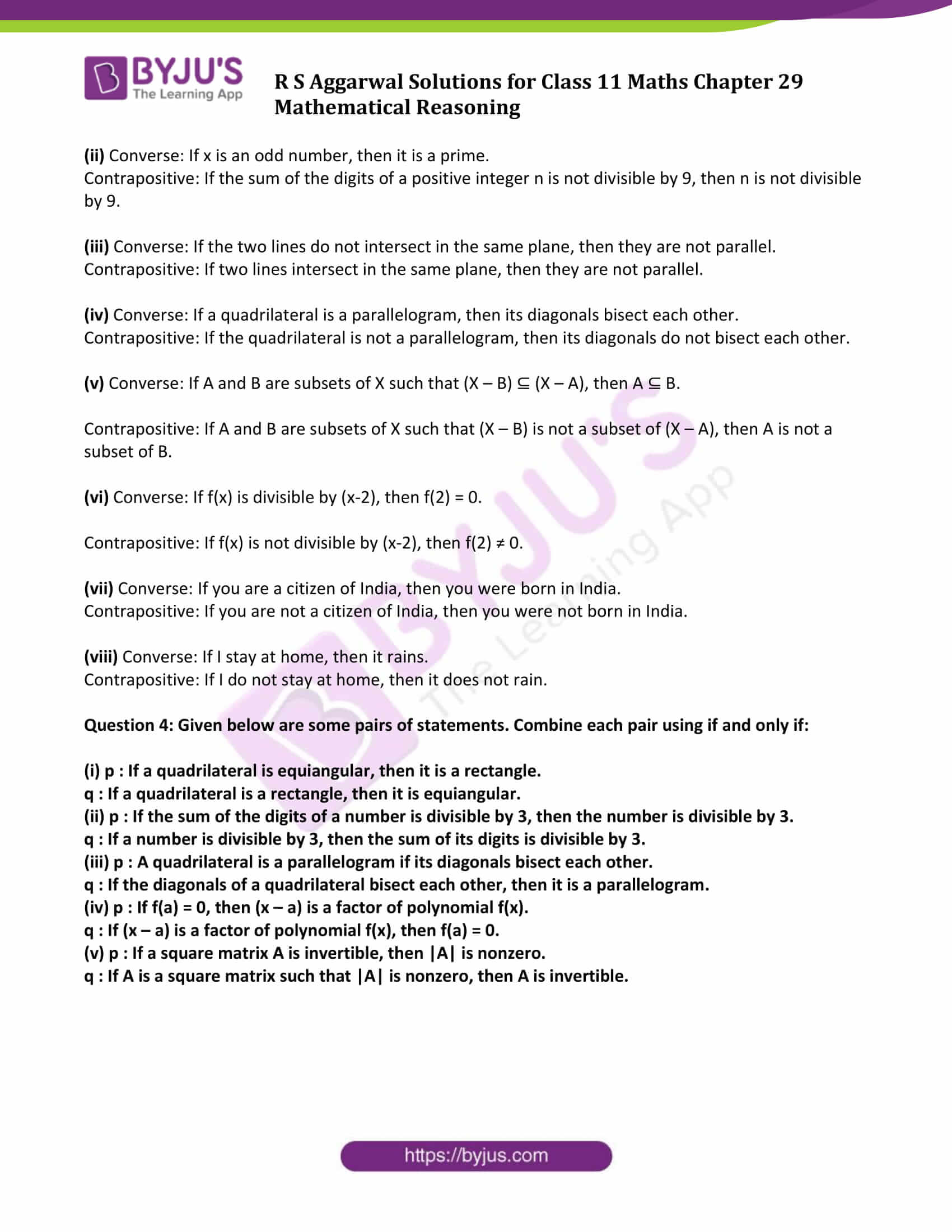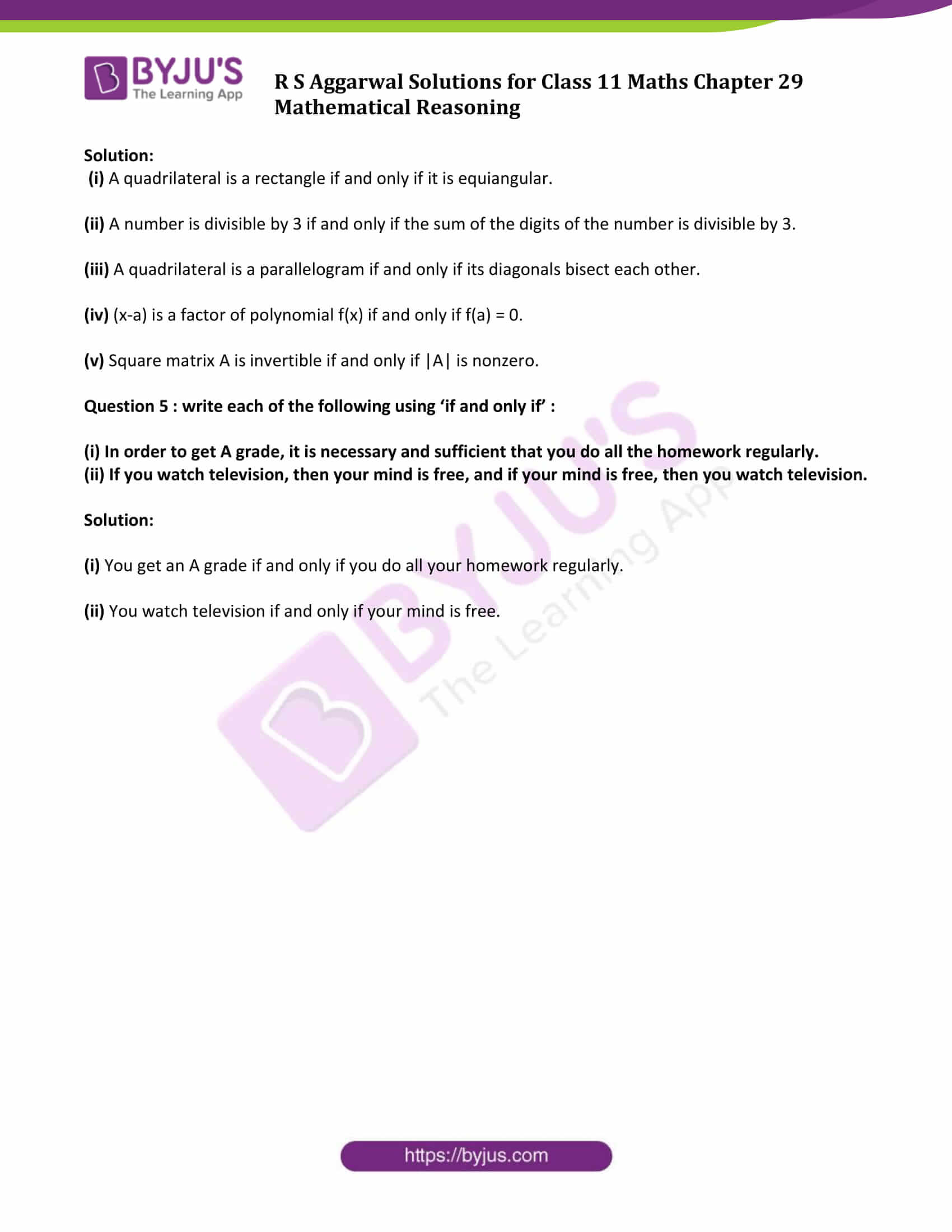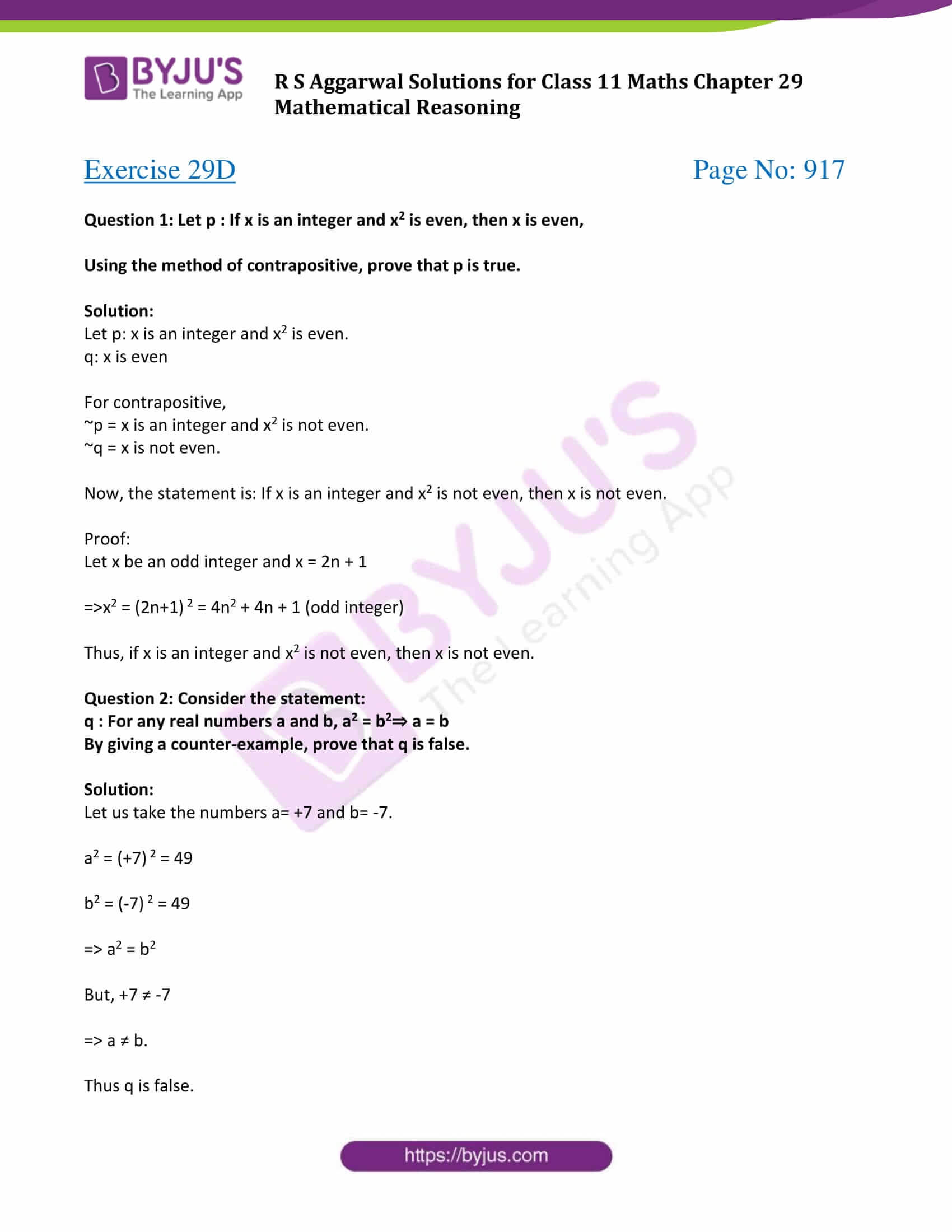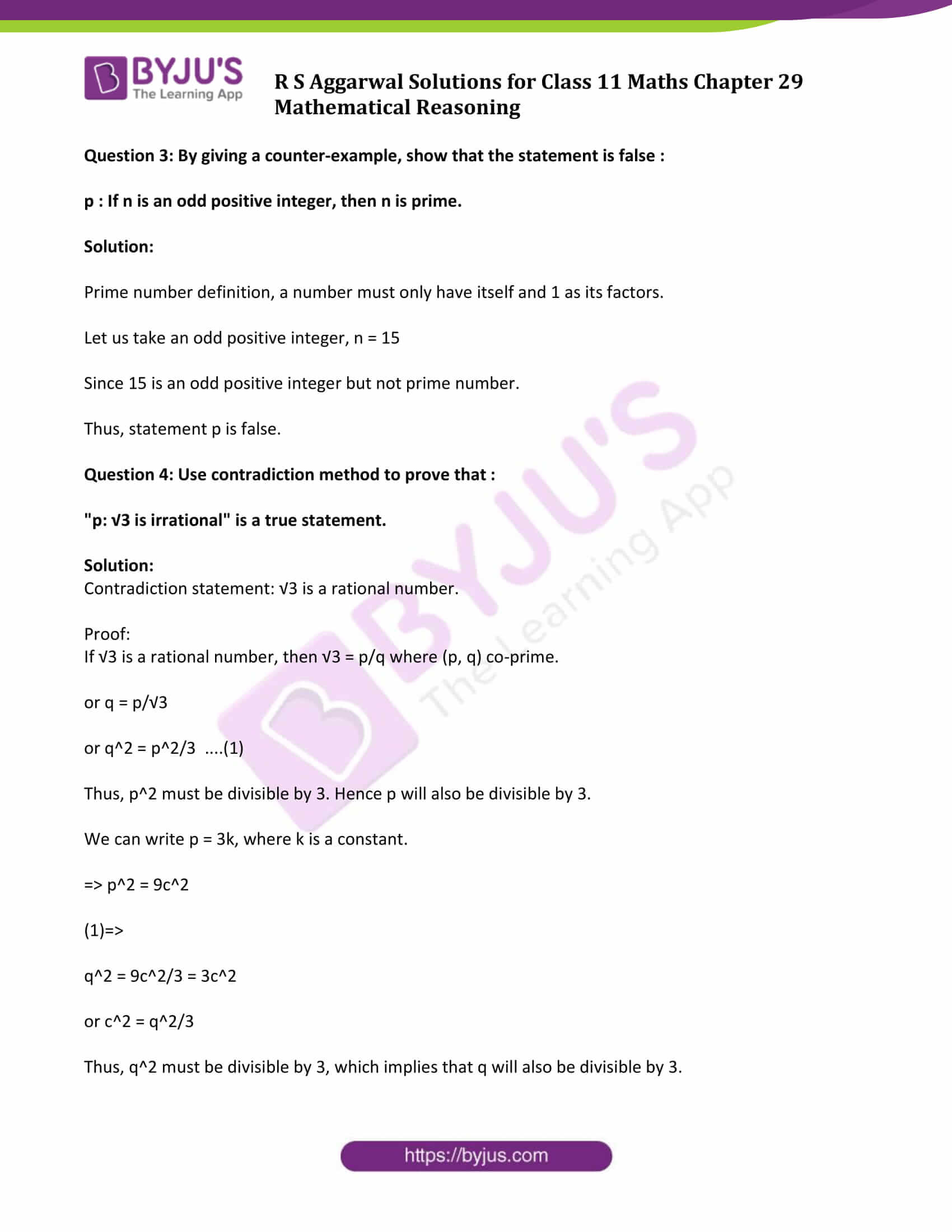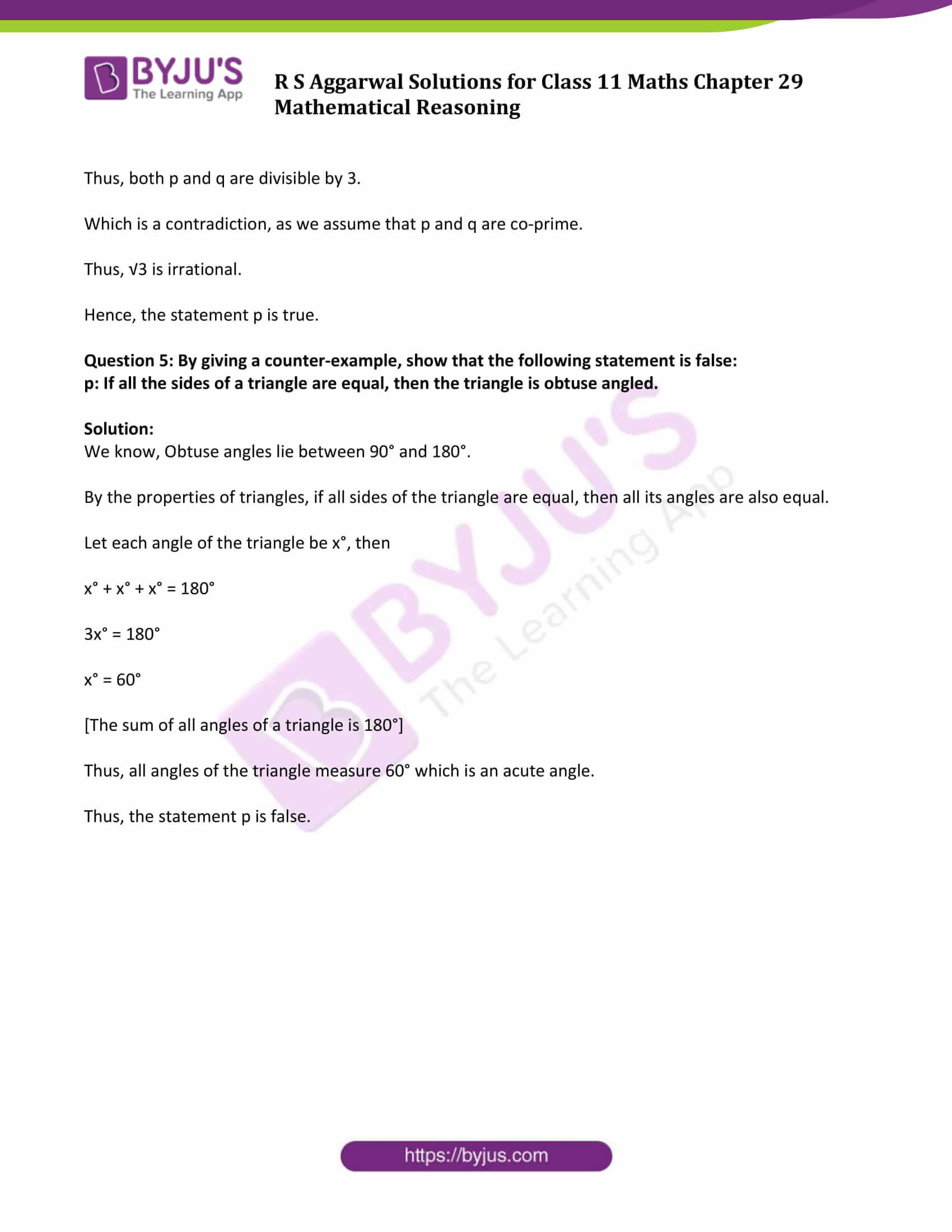### Access Answers to Maths R S Aggarwal Chapter 29 Mathematical Reasoning

Exercise 29A Page No: 898

Question 1: Which of the following sentences are statements? In case of a statement mention whether it is true or false.

(i) The sun is a star.

(ii) √7 is an irrational number.

(iii) The sum of 5 and 6 is less than 10.

(v) Ice is always cold.

(vi) Have you ever seen the Red Fort?

(vii) Every relation is a function.

(viii) The sum of any two sides of a triangle is always greater than the third side.

(ix) May God bless you!

Solution:

(i) The sun is a star is a statement.

It is a scientifically proven fact, therefore this sentence is always true.

(ii) An irrational number is any number which cannot be expressed as a fraction of two integers.

Here, √7 cannot be expressed as a fraction of two integers, so √7 is an irrational number.

Therefore, “√7 is an irrational number” is a statement, and it is true.

(iii) Sum of 5 and 6 = 5 + 6 = 11 > 10

Sum of 5 and 6 is 11, which is greater than 10.

Therefore, “The sum of 5 and 6 is less than 10” is a statement, but not true.

(iv) The sentence ‘Go to your class’ is an order.

This is an Imperative sentence. Hence it is not a statement.

(v) Ice is always cold is a statement.

It is scientifically proven the fact, therefore the sentence is always true.

(vi) The sentence ‘Have you ever seen the Red Fort?

This is an interrogative sentence. Hence not a statement.

(vii) ‘Every relation is a function’ is a statement.

There are relations which are not functions.

Therefore, the sentence is false.

(viii) ‘The sum of any two sides of a triangle is always greater than the third side’

It is a statement and mathematically proven result.

Hence the statement is true.

(ix) ‘May God bless you!’ is an exclamation sentence. Hence it is not a statement.

Question 2: Which of the following sentences are statements? In case of a statement, mention whether it is true or false.

(i) Paris is in France.

(ii) Each prime number has exactly two factors.

(iii) The equation x2 + 5|x| + 6 = 0 has no real roots.

(iv) (2 + √3) is a complex number.

(v) Is 6 a positive integer?

(vi) The product of -3 and -2 is -6.

(vii) The angles opposite the equal sides of an isosceles triangle are equal.

(viii) Oh! it is too hot.

(ix) Monika is a beautiful girl.

(x) Every quadratic equation has at least one real root.

Solution:

(i) Paris is in France, is a statement.

Paris is located in France, so the sentence is true.

So, the statement is true.

(ii) Each prime number has exactly two factors, is a statement.

This is a mathematically proven fact.

So, the statement is true.

(iii) The equation x2 + 5|x| + 6 = 0 has no real roots.

Find the roots of x2 + 5|x| + 6 = 0:

Case 1: x ≥ 0

x2 + 5x + 6 = (x+2)(x+3) = 0 ⇒ x= −2, −3 but we already assumed x ≥ 0, which is a contradiction.

Case 2: x < 0

x2 − 5x + 6 = (x−2)(x−3) = 0 ⇒ x = (2,3) but we already assumed x < 0, which is a contradiction.

So, equation x2 + 5|x| + 6 = 0 has no real roots.

Therefore, the given sentence is true, and it is a statement.

(iv) (2 + √3) is a complex number, is a statement.

Complex numbers are in the form ‘a+ib’.

(2 + √3) cannot be expressed in ‘a+ib’ form,.

2 + √3 is not a complex number.

The given sentence is a statement, and it is false.

(v) Is 6 a positive integer?

This is an interrogative sentence, so it is not a statement.

(vi) The product of -3 and -2 is -6, is a statement.

Product of -3 and -2 = -3 x -2 = 6 ≠ -6

This statement is false.

(vii) The angles opposite the equal sides of an isosceles triangle are equal, is a statement.

It is a mathematically proven result.

So the given sentence is true.

(viii) Oh! it is too hot.

This is an exclamatory sentence, so it is not a statement.

(ix) Monika is a beautiful girl, is not a statement.

The given sentence is an opinion, can be true for some cases, false for some other case.

(x) Every quadratic equation has at least one real root, is a statement.

Because not every quadratic equation will have a real root.

So the given sentence is false.

Question 3: Which of the following statements are true and which are false? In each case give a valid reason for your answer.

(i) p: √11 is an irrational number.

(ii) q: Circle is a particular case of an ellipse.

(iii) r: Each radius of a circle is a chord of the circle.

(iv) S: The center of a circle bisects each chord of the circle.

(v) t: If a and b are integers such that a < b, then –a > -b.

(vi) y: The quadratic equation x2 + x + 1 = 0 has no real roots.

Solution:

(i) p: √11 is an irrational number.

True statement.

Reason:

An irrational number is any number which cannot be expressed as a fraction of two integers.

√11 cannot be expressed as a fraction of two integers, so √11 is an irrational number.

(ii) q: Circle is a particular case of an ellipse.

True statement.

Reason:

The equation of an ellipse is x2/a2 + y2/b2 = 1

Special case: When a = b

Then x2 + y2 = 1, which is an equation of circle.

So, we can say that, a circle is a particular case of an ellipse with the same radius in all points.

(iii) r: Each radius of a circle is a chord of the circle.

False statement.

Reason:

A chord intersects the circle at two points, but radius intersects the circle only at one point.

So the radius is not a chord of the circle.

(iv) S: The center of a circle bisects each chord of the circle.

False statement.

Reason:

The only diameter of a circle is bisected by the center of the circle. Except for diameter, no other chords are passes through the center of a circle.

(v) t: If a and b are integers such that a < b, then –a > -b.

True statement.

Reason:

a < b, then –a > -b [By rule of inequality]

(vi) y: The quadratic equation x2 + x + 1 = 0 has no real roots.

True statement.

Reason:

General form of a quadratic equation, ax2 + bx + c = 0, has no real roots if discriminant, D < 0.

Where D= b2 – 4ac < 0.

Given equation; x2 + x + 1 = 0

Here, a= 1, b = 1 and c = 1

Now, b2 – 4ac = 1 – 4 x 1 x 1 = -3 < 0

So, there is no real root.

Exercise 29B Page No: 904

Question 1: Split each of the following into simple sentences and determine whether it is true or false.

(i) A line is straight and extends indefinitely in both the directions.

(ii) A point occupies a position and its location can be determined.

(iii) The sand heats up quickly in the sun and does not cool down fast at night.

(iv) 32 is divisible by 8 and 12.

(v) x = 1 and x = 2 are the roots of the equation x2 – x – 2 = 0.

(vi) 3 is rational, and √3 is irrational.

(vii) All integers are rational numbers and all rational numbers are not real numbers.

(viii) Lucknow is in Uttar Pradesh, and Kanpur is in Uttarakhand.

Solution:

(i) Let p: A line is straight.

And q: A line extends indefinitely in both the directions.

Both the sentences are True.

Therefore, the given sentence is TRUE.

(ii) Let p: A point occupies a position.

And q: Its location can be determined.

Both the sentences are True.

Therefore, the given sentence is TRUE.

(iii) Let p: The sand heats up quickly in the sun.

And q: The sand does not cool down fast at night.

Both the sentences are True.

Therefore, the given sentence is TRUE.

(iv) Let p: 32 is divisible by 8.

And q: 32 is divisible by 12.

The first sentence is True and the second sentence is False.

Therefore, the given sentence is FALSE.

(v) Let p: x = 1 is a root of the equation x2 – x – 2 = 0

And q: x = 2 is a root of the equation x2 – x – 2 = 0

The first sentence is False and the second sentence is True.

Therefore, the given sentence is FALSE.

(vi) Let p: 3 is rational.

And, q: √3 is irrational.

Both the sentences are True.

Therefore, the given sentence is TRUE.

(vii) Let p: All integers are rational numbers.

And, q: All rational numbers are not real numbers.

The first sentence is True and the second sentence is False.

Therefore, the given sentence is FALSE.

(viii) Let p: Lucknow is in Uttar Pradesh.

And q: Kanpur is in Uttarakhand.

The first sentence is True and the second sentence is False.

Therefore, the given sentence is FALSE.

Question 2: Split each of the following into simple sentences and determine whether it is true or false. Also, determine whether an ‘inclusive or’ or ‘exclusive or’ is used.

(i) The sum of 3 and 7 is 10 or 11.

(ii) (1 + i) is a real or a complex number.

(iii) Every quadratic equation has one or two real roots.

(iv) You are wet when it rains, or you are in a river.

(v) 24 is a multiple of 5 or 8.

(vi) Every integer is rational or irrational.

(vii) For getting a driving license, you should have a ration card or a passport.

(viii) 100 is a multiple of 6 or 8.

(ix) Square of an integer is positive or negative.

(x) Sun rises or Moon sets.

Solution:

(i) The sum of 3 and 7 is 10 or 11.

Let p: The sum of 3 and 7 is 10.

And q: The sum of 3 and 7 is 11.

First sentence is TRUE. Second sentence is FALSE.

Or used is ‘Exclusive or’.

(ii) (1 + i) is a real or a complex number.

Let q: (1 + i) is a real number.

And q: (1 + i) is a complex number.

First sentence is TRUE. Second sentence is FALSE.

Or used is ‘Exclusive or’.

(iii) Every quadratic equation has one or two real roots.

Let q: Every quadratic equation has one real root.

And q: Every quadratic equation has two real roots.

P and q both are False.

Given sentence is FALSE.

(iv) You are wet when it rains, or you are in a river.

Let p: You are wet when it rains.

And q: You are wet when you are in a river.

(p or q) is true.

Or used is ‘Inclusive or’ because you can get wet either it rains or when you are in the river.

(v) 24 is a multiple of 5 or 8.

Let p: 24 is a multiple of 5.

And q: 24 is a multiple of 8.

First sentence is FALSE. Second sentence is TRUE.

Or used is ‘Exclusive or’.

(vi) Every integer is rational or irrational.

Let p: Every integer is rational.

And q: Every integer is irrational.

(p or q) is true.

‘Or’ used is ‘Exclusive or’.

(vii) For getting a driving license you should have a ration card or a passport.

Let p: For getting a driving license you should have a ration card.

And q: For getting a driving license you should have a passport.

(p or q) is true.

Or used is ‘Inclusive or’, because you can get a driving license with ration card or with passport or when they have both.

(viii) 100 is a multiple of 6 or 8.

Let p: 100 is a multiple of 6.

And q: 100 is a multiple of 8.

(p or q) is FALSE.

(ix) Square of an integer is positive or negative.

Let p: Square of an integer is positive.

And q: Square of an integer is negative.

(p or q) is FALSE.

(x) Sun rises or Moon sets.

Let p: Sun rises.

And q: Moon sets.

(p or q) is TRUE.

Here, Or used is ‘Exclusive or’.

Question 3: Find the truth set in case of each of the following open sentences defined on N:

(i) x + 2 < 10

(ii) x + 5 < 4

(iii) x + 3 > 2

Solution:

(i)

Given: The open sentence x + 2 < 10 is defined on N.

Here N: {1, 2, 3, 4, 5, 6, 7, 8, …….…}

At x = 1 ⇒ x + 2 = 3 < 10

At x = 2 ⇒ x + 2 = 4 < 10

At x = 3 ⇒ x + 2 = 5 < 10

At x = 4 ⇒ x + 2 = 6 < 10

At x = 5 ⇒ x + 2 = 7 < 10

At x = 6 ⇒ x + 2 = 8 < 10

At x = 7 ⇒ x + 2 = 9 < 10

At x = 8 ⇒ x + 2 = 10

x = {1, 2, 3, 4, 5, 6, 7} satisfies x + 2 <10.

So, the truth set of open sentence x + 2 < 10 defined on N : {1, 2, 3, 4, 5, 6, 7}

(ii) The open sentence x + 5 < 4 is defined on N.

Here N: {1, 2, 3, 4, 5, 6, 7, 8, …….…}

At x = 1 ⇒ 1 + 5 = 6 > 4

So, the truth set of open sentence x + 5 < 4 defined on N is an empty set, {}.

(iii) The open sentence x + 3 > 2 is defined on N.

Here N: {1, 2, 3, 4, 5, 6, 7, 8, …….…}

At x = 1 ⇒ x + 3 = 4 > 2

At x = 2 ⇒ x + 3 = 5 > 2

At x = 3 ⇒ x + 3 = 6 > 2

At x = 4 ⇒ x + 3 = 7 > 2

At x = 5 ⇒ x + 3 = 8 > 2

At x = 6 ⇒ x + 3 = 9 > 2

At x = 7 ⇒ x + 3 = 10 > 2

And so on…

x = {1, 2, 3, 4, 5, 6, 7….} satisfies x + 3 > 2.

So, the truth set of open sentence x + 3 > 2 defined on N is an infinite set i.e. {1, 2, 3, 4, 5, 6, 7, ………}

Question 4: Let A = {2, 3, 5, 7}. Examine whether the statements given below are true or false.

(i) ∃ x ∈ A such that x + 3 > 9.

(ii) ∃ x ∈ A such that x is even.

(iii) ∃ x ∈ A such that x + 2 = 6.

(iv) ∀ x ∈ A, x is prime.

(v) ∀ x ∈ A, x + 2 < 10.

(vi) ∀ x ∈ A, x + 4 ≥ 11

Solution:

Given: A = {2, 3, 5, 7}

(i) ∃ x ∈ A such that x + 3 > 9.

We have to check whether there exists ‘x’ which belongs to ‘A’, such that x + 3 > 9.

When x = 7 ∈ A,

x + 3 = 7 + 3 = 10 > 9

So, ∃ x ∈ A and x + 3 > 9.

So, the given statement is TRUE.

(ii) ∃ x ∈ A such that x is even.

We have to check whether there exists ‘x’ which belongs to ‘A’, such that x is even.

x = 2, is an even number and 2 ∈ A.

So, the given statement is TRUE.

(iii) ∃ x ∈ A such that x + 2 = 6.

We have to check whether there exists ‘x’ which belongs to ‘A’, such that x + 2 = 6.

At x = 2 ⇒ x + 2 = 4 ≠ 6

At x = 3 ⇒ x + 2 = 5 ≠ 6

At x = 5 ⇒ x + 2 = 7 ≠ 6

At x = 7 ⇒ x + 2 = 9 ≠ 6

None of the values satisfy the equation.

So, the given statement is FALSE.

(iv) ∀ x ∈ A, x is prime.

We have to check whether for all ‘x’ which belongs to ‘A’, such that x is a prime number.

All ‘x’ which belongs to A = {2, 3, 5, 7} is a prime number.

All are prime numbers.

So, the given statement is TRUE.

(v) ∀ x ∈ A, x + 2 < 10.

We have to check whether for all ‘x’ which belongs to ‘A’, such that x + 2 < 10.

A = {2, 3, 5, 7}

At x = 2 ⇒ x + 2 = 4 < 10

At x = 3 ⇒ x + 2 = 5 < 10

At x = 5 ⇒ x + 2 = 7 < 10

At x = 7 ⇒ x + 2 = 9 < 10

∀ x ∈ A, x + 2 < 10, is a TRUE statement.

(vi) ∀ x ∈ A, x + 4 ≥ 11.

We have to check whether for all ‘x’ which belongs to ‘A’, such that x + 4 ≥ 11.

A = {2, 3, 5, 7}

At x = 2 ⇒ x + 4 = 6 ≥ 11

At x = 3 ⇒ x + 4 = 7 ≥ 11

At x = 5 ⇒ x + 4 = 9 ≥ 11

At x = 7 ⇒ x + 4 = 11 ≥ 11

Only for x = 7, statement is true.

∀ x ∈ A, x + 4 ≥ 11, is a FALSE statement.

Exercise 29C Page No: 911

Question 1: Rewrite the following statement in five different ways conveying the same meaning.

If a given number is a multiple of 6, then it is a multiple of 3.

Solution:

(i) A given number is a multiple of 6, it implies that it is a multiple of 3 as well.

(ii) For a given number to be a multiple of 6, it is necessary that it is a multiple of 3.

(iii) A given number is a multiple of 6 only if it is a multiple of 3.

(iv) If the given number in not a multiple of 3, then it is not a multiple of 6.

(v) For a given number to be a multiple of 3, it is sufficient that the number is multiple of 6.

Question 2: Write each of the following statements in the form ‘if …. then’ :

(i) A rhombus is a square only if each of its angles measures 900.

(ii) When a number is a multiple of 9, it is necessarily a multiple of 3.

(iii) You get a job implies that your credentials are good.

(iv) Atmospheric humidity increase only if it rains.

(v) If a number is not a multiple of 3, then it is not a multiple of 6.

Solution:

(i) If each of the angles of a rhombus measures 90°, then the rhombus is a square.

(ii) If a number is a multiple of 9, then the number is multiple of 3.

(iii) If you get a job, then your credentials are good.

(iv) If it rains, then the atmospheric humidity increases.

(v) If a number is a multiple of 6, then it is a multiple of 3.

Question 3: Write the converse and contrapositive of each of the following :

(i) If x is a prime number, then x is odd.

(ii) If a positive integer n is divisible by 9, then the sum of its digits is divisible by 9.

(iii) If the two lines are parallel, then they do not intersect in the same plane.

(iv) If the diagonal of a quadrilateral bisect each other, then it is a parallelogram.

(v) If A and B are subsets of X such that A ⊆ B, then (X – B) ⊆ (X – A)

(vi) If f(2) = 0, then f(x) is divisible by (x – 2).

(vii) If you were born in India, then you are a citizen of India.

(viii) If it rains, then I stay at home.

Solution:

(i) Converse: If x is an odd number, then it is a prime.

Contrapositive: If x is not an odd number, then it is not a prime.

(ii) Converse: If x is an odd number, then it is a prime.

Contrapositive: If the sum of the digits of a positive integer n is not divisible by 9, then n is not divisible by 9.

(iii) Converse: If the two lines do not intersect in the same plane, then they are not parallel.

Contrapositive: If two lines intersect in the same plane, then they are not parallel.

(iv) Converse: If a quadrilateral is a parallelogram, then its diagonals bisect each other.

Contrapositive: If the quadrilateral is not a parallelogram, then its diagonals do not bisect each other.

(v) Converse: If A and B are subsets of X such that (X – B) ⊆ (X – A), then A ⊆ B.

Contrapositive: If A and B are subsets of X such that (X – B) is not a subset of (X – A), then A is not a subset of B.

(vi) Converse: If f(x) is divisible by (x-2), then f(2) = 0.

Contrapositive: If f(x) is not divisible by (x-2), then f(2) ≠ 0.

(vii) Converse: If you are a citizen of India, then you were born in India.

Contrapositive: If you are not a citizen of India, then you were not born in India.

(viii) Converse: If I stay at home, then it rains.

Contrapositive: If I do not stay at home, then it does not rain.

Question 4: Given below are some pairs of statements. Combine each pair using if and only if:

(i) p : If a quadrilateral is equiangular, then it is a rectangle.

q : If a quadrilateral is a rectangle, then it is equiangular.

(ii) p : If the sum of the digits of a number is divisible by 3, then the number is divisible by 3.

q : If a number is divisible by 3, then the sum of its digits is divisible by 3.

(iii) p : A quadrilateral is a parallelogram if its diagonals bisect each other.

q : If the diagonals of a quadrilateral bisect each other, then it is a parallelogram.

(iv) p : If f(a) = 0, then (x – a) is a factor of polynomial f(x).

q : If (x – a) is a factor of polynomial f(x), then f(a) = 0.

(v) p : If a square matrix A is invertible, then |A| is nonzero.

q : If A is a square matrix such that |A| is nonzero, then A is invertible.

Solution:

(i) A quadrilateral is a rectangle if and only if it is equiangular.

(ii) A number is divisible by 3 if and only if the sum of the digits of the number is divisible by 3.

(iii) A quadrilateral is a parallelogram if and only if its diagonals bisect each other.

(iv) (x-a) is a factor of polynomial f(x) if and only if f(a) = 0.

(v) Square matrix A is invertible if and only if |A| is nonzero.

Question 5 : write each of the following using ‘if and only if’ :

(i) In order to get A grade, it is necessary and sufficient that you do all the homework regularly.

(ii) If you watch television, then your mind is free, and if your mind is free, then you watch television.

Solution:

(i) You get an A grade if and only if you do all your homework regularly.

(ii) You watch television if and only if your mind is free.

Exercise 29D Page No: 917

Question 1: Let p : If x is an integer and x2 is even, then x is even,

Using the method of contrapositive, prove that p is true.

Solution:

Let p: x is an integer and x2 is even.

q: x is even

For contrapositive,

~p = x is an integer and x2 is not even.

~q = x is not even.

Now, the statement is: If x is an integer and x2 is not even, then x is not even.

Proof:

Let x be an odd integer and x = 2n + 1

⇒x2 = (2n+1) 2 = 4n2 + 4n + 1 (odd integer)

Thus, if x is an integer and x2 is not even, then x is not even.

Question 2: Consider the statement:

q : For any real numbers a and b, a2 = b2⇒ a = b

By giving a counter-example, prove that q is false.

Solution:

Let us take the numbers a= +7 and b= -7.

a2 = (+7) 2 = 49

b2 = (-7) 2 = 49

⇒ a2 = b2

But, +7 ≠ -7

⇒ a ≠ b.

Thus q is false.

Question 3: By giving a counter-example, show that the statement is false :

p : If n is an odd positive integer, then n is prime.

Solution:

Prime number definition, a number must only have itself and 1 as its factors.

Let us take an odd positive integer, n = 15

Since 15 is an odd positive integer but not prime number.

Thus, statement p is false.

Question 4: Use contradiction method to prove that :

“p: √3 is irrational” is a true statement.

Solution:

Contradiction statement: √3 is a rational number.

Proof:

If √3 is a rational number, then √3 = p/q where (p, q) co-prime.

or q = p/√3

or q^2 = p^2/3 ….(1)

Thus, p^2 must be divisible by 3. Hence p will also be divisible by 3.

We can write p = 3k, where k is a constant.

⇒ p^2 = 9c^2

(1)⇒

q^2 = 9c^2/3 = 3c^2

or c^2 = q^2/3

Thus, q^2 must be divisible by 3, which implies that q will also be divisible by 3.

Thus, both p and q are divisible by 3.

Which is a contradiction, as we assume that p and q are co-prime.

Thus, √3 is irrational.

Hence, the statement p is true.

Question 5: By giving a counter-example, show that the following statement is false:

p: If all the sides of a triangle are equal, then the triangle is obtuse angled.

Solution:

We know, Obtuse angles lie between 90° and 180°.

By the properties of triangles, if all sides of the triangle are equal, then all its angles are also equal.

Let each angle of the triangle be x°, then

x° + x° + x° = 180°

3x° = 180°

x° = 60°

[The sum of all angles of a triangle is 180°]

Thus, all angles of the triangle measure 60° which is an acute angle.

Thus, the statement p is false.

## R S Aggarwal Solutions For Class 11 Maths Chapter 29 Exercises:

Get detailed solutions for all the questions listed under below exercises:

Exercise 29A Solutions

Exercise 29B Solutions

Exercise 29C Solutions

Exercise 29D Solutions

## R S Aggarwal Solutions for Chapter 29 Mathematical Reasoning

R S Aggarwal Chapter 29 – Mathematical Reasoning, contains the answers to all the questions listed in this chapter. Let us have a look at some of the topics covered here:

• Mathematical Reasoning Introduction
• Sentences Classification
• Statements
• Logical Connectives
• Compounding of sentences
• Quantifiers and Quantified statements
• Conditional and Biconditional statements
• Validating Statements